Introduction to Digital Circuits : 2 of 3

In this session, Part 2 of Digital Circuits, we will look at Digital ICs and Combinational Logic

## What are Digital ICs?

The abbreviation IC stands for "integrated circuit" and in practice denotes any semiconductor-based chip comprising an integrated set of digital circuitry. Digital ICs come in many different types; the following listing shows the IC types used for various applications.

Microcomputers
ICs that carry out various types of processing
Memory
ICs that store data
Standard Logic ICs
ICs put together in various combinations to implement particular functionalities
Custom Logic ICs
Original dedicated circuitry implemented by or for the needs of a particular user

A standard logic IC is a single, small, integrated package carrying basic components and common functionalities for a logic circuit. These ICs are core components of logic circuits. Today's session on digital ICs will focus on this type of IC.

## Standard Logic ICs: the Foundation of Digital Circuitry

There are roughly 600 types of standard logic ICs, from basic chips to highly functional arithmetic-and-logic units. There are two different types of implementation: TTL and CMOS.

TTL ICs
Transistor-transistor logic ICs: The main circuitry is built with bipolar transistors. These chips run on 5-V power.
CMOS ICs
Complementary metal oxide semiconductor ICs: The main circuitry is built from pairs of p-type and n-type metal oxide semiconductor field-effect transistors (MOSFETs). Voltages used to drive these chips extend over a wide range.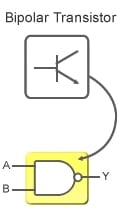Figure 1: TTL IC

If a design calls for signals to be passed among digital ICs, then the designer must know the logical conditions that will produce an H or L, and the voltage ranges that represent these conditions. Voltage ranges corresponding to logical conditions are called logic levels. Communicating ICs must use the same logic levels; use of different levels will disable communication and may even cause IC breakage.

By convention, TTL ICs use the following levels.

Input Signals
0 V to 0.8 V is L; 2.0 V and up is H
Output signals
0 V to 0.4 V is L; 2.4 V and up is H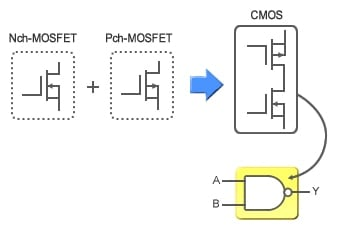Figure 2: CMOS IC

TTL IC makers must design their ICs to meet the TTL interface standards indicated above. Since these values are now standard, logic designers working with TTL IC communication need not concern themselves with logic levels.

The case is different for CMOS ICs, where there are many different series with different logic levels, and where logic levels may also differ according to the supplied voltage. This means that designers must take care to use consistent logic levels when connecting different CMOS ICs.

## The Significance of Fan-Out

When connecting standard logic ICs, care must be taken to avoid connecting too many ICs to a given output. With TTL ICs, the output current limits the number of IC connections. The term fan-out denotes the maximum number of ICs that an output can connect to.

Remember that TTL ICs consist chiefly of bipolar transistors; current is therefore required to execute switching. The fan-out of a TTL IC is the ratio of its output current to the current used by each input (see Fig. 3). If fan-out is exceeded, there is a risk that the output may be unable to maintain proper output logic levels.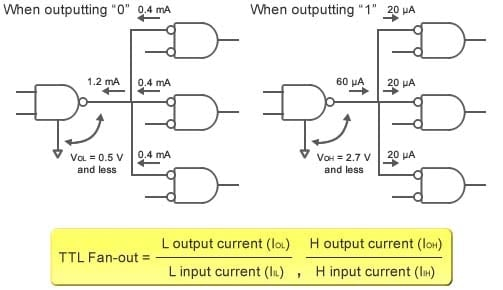Figure 3: TTL IC Fan-out

In the case of CMOS ICs, where very little current flows to the input terminals, fan-out cannot be determined by looking at currents. Instead, fan-out is determined by load capacity. (See Fig. 4.) The load capacities indicated on CMOS IC data sheets are found by measuring the propagation delay. If load capacity is exceeded, propagation delay may lengthen to the point where malfunction occurs.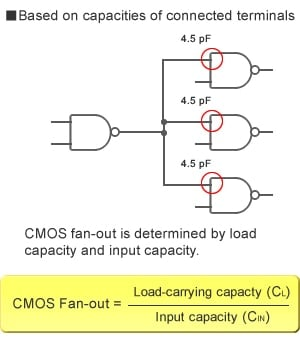Figure 4: CMOS IC Fan-out

### Open Drains: Wiring Outputs Together

In an open drain configuration, there is no MOSFET at the VCC side of the output stage (Fig. A, left) of a general-purpose CMOS IC, and so the output cannot go High (Fig. A, right). The output will be either Low or high-impedance (where the output pin is disconnected from the circuit and unable to output a voltage or current).

The high-impedance state is unstable, as the output level is indeterminate. For this reason, the output connects to power through an interposed pull-up resistor, and fixed at High. Note that the output connected to the pull-up resistor need not be at the same voltage as the power voltage. This makes it possible to connect ICs having different logic levels.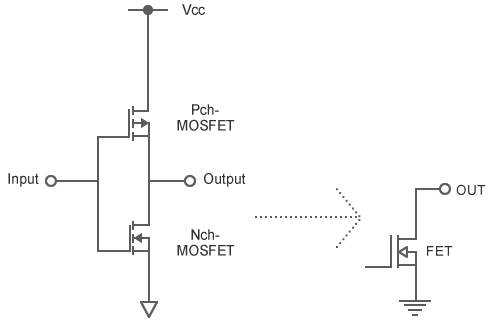Figure A: CMOS output and Open Drain output

## Combinational Logic

Combinational logic refers to digital circuitry whose output is a function of the present input signals only. Sequential logic, in contrast, is not determined solely by present inputs, but also by internal memory circuitry and synchronous circuitry.

In today's session, let's look at combinational logic.

A combinational logic circuit is a series of logic gates: AND, OR, NOT, XOR, and others. (We covered logic gates in a previous session.) Correct combinations of logic gates can be used to implement a wide variety of functions. Let's look at two common types of combinational logic: a multiplexer, and a decoder.

## Selecting Output Signals with a Multiplexer

A multiplexer is a signal switcher that selects an output signal from among multiple input signals. Its operation resembles that of a vending machine: press one of many available buttons, and the selected product appears at the machine's single outlet.

We can represent the action of a multiplexer as a series of switches, as shown in Fig.5. Switch A and Switch B each consist of a bank of four internal switches. If Switch A and Switch B are both set to 0, input 0 will connect to the output, as you can see from the figure. In other words, the signal carried by input 0 will propagate through the output, while other inputs will be blocked. Similarly, {A = 1 and B = 0} selects input 1 for output; {A = 0 and B = 1} selects input 2, and {A = 1 and B = 1} selects input 3. As you can see, then, the two switches A and B implement a multiplexer that can be used to select and propagate any one of four inputs.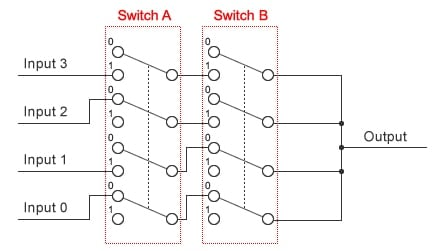Figure 5: Multiplexer: A Combination of Switches

Figure 6 shows how this multiplexer can be represented in terms of combinational logic. As you can see, the multiplexer can be built entirely from AND and OR gates. Judgments made by the AND section are narrowed down to one by the OR gate.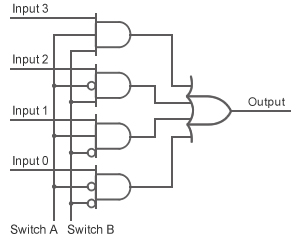Figure 6: Multiplexer Implemented by Combinational Logic

## Decoder: Decoding the Input

A decoder is a combinational logic circuit that turns one of several outputs ON (High) based on the logic of the inputs. Figure 7 represents a decoder in terms of combinational logic.

Figure 8 shows a truth table for this decoder. The table shows that the values of the two input signals uniquely select one of the four available output lines for actual output. It's worth noting that if we consider the input pair as a binary value, and the four output lines as the decimal values 0, 1, 2, and 3, then we can say that this circuit is a decoder, as its function is to input a binary value and output a decimal value.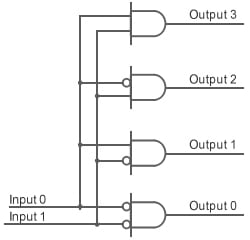Figure 7: Decoder Implemented by Combinational Logic

Input1 Input0 Output3 Output2 Output１ Output0
0 0 0 0 0 1
0 1 0 0 1 0
1 0 0 1 0 0
1 1 1 0 0 0

Figure 8: Truth Table of a Decoder

In addition to decoders, combinational logic circuitry can be used to create comparators, full adders, half adders, multipliers, subtracters, barrel shifters, and other functionalities. Most of these functionalities can be implemented using only multiplexers and decoders of the type described above. Even so, circuits built from these constituents alone are likely to include redundancies and experience various other problems. These circuits must therefore be simplified and compressed.

In this session we looked at combinational logic. In our next session we will look at sequential logic.

## Module List

1. Elemental Digital Circuits
2. Digital ICs/Combinational Logic
3. Sequential Logic